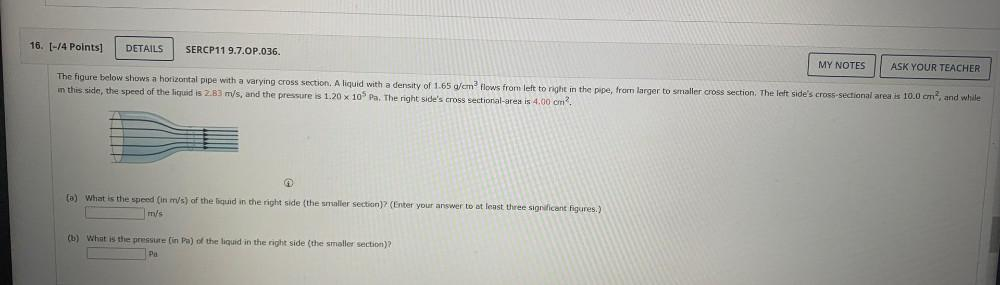Question:

# The figure below shows a horizontal pipe with a varying

Last updated: 7/25/2022The figure below shows a horizontal pipe with a varying cross section. A liquid with a density of 1.65 g/cm³ flows from left to right in the pipe, from larger to smaller cross section. The left side's cross-sectional area is 10.0 cm², and while in this side, the speed of the liquid is 2.83 m/s, and the pressure is 1.20*10⁵ Pa. The right side's cross sectional-area is 4.00 cm². (a) What is the speed (in m/s) of the liquid in the right side (the smaller section)? (Enter your answer to at least three significant figures.) (b) What is the pressure (in Pa) of the liquid in the right side (the smaller section)?